﻿ Year 3 Number and Place Value (1)
 HOME ABOUT US SHOP PRIVACY CONTACT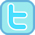Year 1 Year 2 Year 3 Year 4 Year 5 Year 6

# Year 3 Number and Place Value (1)

### Pupils should be taught to count from 0 in multiples of 4, 8, 50 and 100; find 10 or 100 more or less than a given number.

 Our printable resources...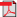Counting in 4s Mad Maths Minutes Sets A & B          Sets C & D          Sets A & B (black)          Sets C & D (black)Counting in 8s Mad Maths Minutes Sets A & B          Sets C & D          Sets A & B (black)          Sets C & D (black)Counting in 50s Mad Maths Minutes Sets A & B          Sets C & D          Sets A & B (black)          Sets C & D (black)Adding 10 to 2-digit Mad Maths Minutes Sets A & B          Sets C & D          Sets A & B (black)          Sets C & D (black)Adding 10 to 2-digit (60 Questions) Sheet 1          Sheet 2          Sheet 3          Sheet 4          Sheet 5Subtract 10 from 2-digit Mad Maths Minutes Sets A & B          Sets C & D          Sets A & B (black)          Sets C & D (black)Subtracting 10 from 2-digit (60 Questions) Sheet 1          Sheet 2          Sheet 3          Sheet 4          Sheet 5Adding / Subtracting 10 (2-digit) Mad Maths Minutes Sets A & B          Sets C & D          Sets A & B (black)          Sets C & D (black)Adding / Subtracting 10 from 2-digit (60 Questions) Sheet 1          Sheet 2          Sheet 3          Sheet 4          Sheet 5Adding/Subtracting 10 to/from 3-digit Mad Maths Minutes Sets A & B          Sets C & D          Sets A & B (black)          Sets C & D (black)Adding 100 to 3-digit Mad Maths Minutes Sets A & B          Sets C & D          Sets A & B (black)          Sets C & D (black)Adding 100 to 3-digit (60 Questions) Sheet 1          Sheet 2          Sheet 3          Sheet 4          Sheet 5Subtract 100 from 3-digit Mad Maths Minutes Sets A & B          Sets C & D          Sets A & B (black)          Sets C & D (black)Subtracting 100 from 3-digit (60 Questions) Sheet 1          Sheet 2          Sheet 3          Sheet 4          Sheet 5Adding/Subtracting 100 to/from 3-digit Mad Maths Minutes Sets A & B          Sets C & D          Sets A & B (black)          Sets C & D (black)Adding/Subtracting 100 to/from 3-digit (60 Questions) Sheet 1          Sheet 2          Sheet 3          Sheet 4          Sheet 5Counting in 4sCounting in 8sOur online activities...Counting in 4s Interactivity (Looped)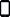Counting in 4s (How far can you go?)Counting in 8s Interactivity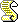Counting in 8s (How far can you go?)Counting in 50s InteractiveCounting in 100s (hundreds) Interactive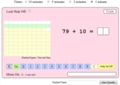Add 10 to 2-digit Alien Attack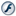Adding 10 to 2-digit Interactive Mad MathsAdd 10 to 2-digit Top of the ClassSubtract 10 from 2-digit Alien AttackSubtracting 10 from 2-digit Numbers Interactive Mad MathsAdding / Subtracting 10 (2-digit) InteractiveAdding 100 to 3-digit Numbers InteractiveSubtract 100 from 3-digit InteractiveAdding/Subtracting 100 to/from 3-digit Numbers